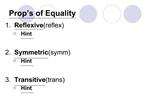DownloadDownload Presentation2.6 Algebraic Proof

2.6 Algebraic Proof

Télécharger la présentation2.6 Algebraic Proof

- - - - - - - - - - - - - - - - - - - - - - - - - - - E N D - - - - - - - - - - - - - - - - - - - - - - - - - - -
Presentation Transcript

1. 2.6 Algebraic Proof

2. Prop�s of Equality 1. Reflexive (reflex) Hint 2. Symmetric (symm) Hint 3. Transitive (trans) Hint

5. Example � Name the prop used: If 3x = 120 ? x = 40. If 12 = AB ? AB = 12. If AB = BC & BC = CD ? AB = CD. If y = 75 & y = m<A ? m<A = 75.

6. Paragraph Proof (review) � informal proof in form of paragraph that uses facts to justify each step 2-Column Proof � formal proof in which 2 columns are needed: 1. statements 2. reasons

7. Parts of 2-Column Proof Given: ~~~~~~~ Prove: ~~~~~~~

8. Example 6-2a

9. Example 6-2c

10. Example 6-2e

11. Example 6-4b

12. Example 6-4c

13. Assignment 2.6 p. 97 (14-25 all, 26-32 even, 38-42 even, 48-52 even)